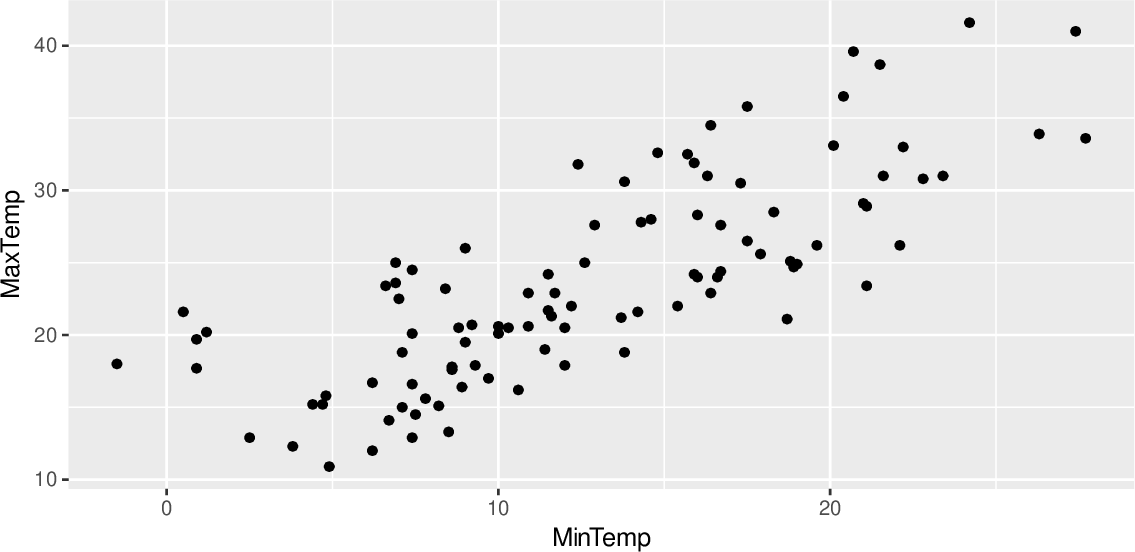Data Science Desktop Survival Guide by Graham WilliamsDesktop Survival Project Home Preface Data Science Introducing R R Constructs R Tasks R Strings R Read, Write, and Create Data Template Data Exploration Data Wrangling Data Visualisation Statistics ML Template ML Scenarios ML Activities ML Applications ML Algorithms Cluster Analysis Decision Trees Computer Vision Graph Data Privacy Literate Data Science Coding with Style Resources Bibliography Index

## Scatter Plot

20200822set.seed(26439) ds %>%   sample_n(100) %>%   ggplot(aes(x=min_temp, y=max_temp)) +   geom_point() +   labs(x = vnames["min_temp"],        y = vnames["max_temp"]) The simplest of plots is a scatter plot which displays a two dimensional plot of points. The dimensions are the x= and y= axes. The observations from the dataset, which are the rows in the dataset, are plotted, scattering the points over the plot. Using the template variable ds the dataset is piped (tidyr::https://www.rdocumentation.org/packages/tidyr/topics/ggplot2::aes() are set up with min_temp as the x= axis and max_temp as the y= axis. To add the points (observations) to the plot we employ ggplot2::geom_point().